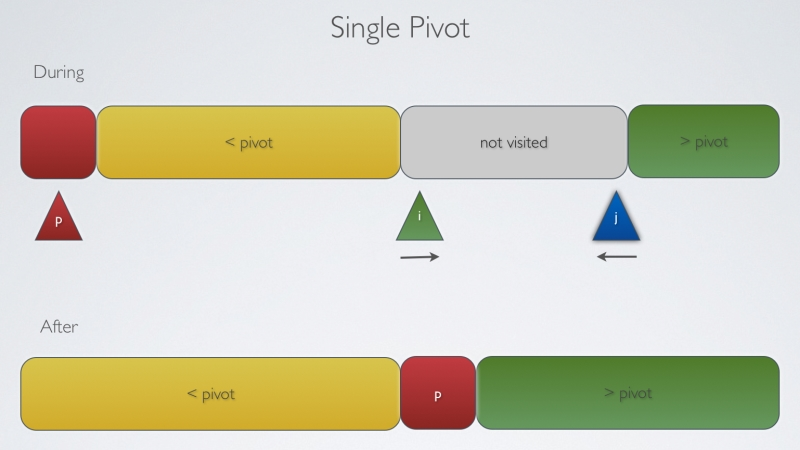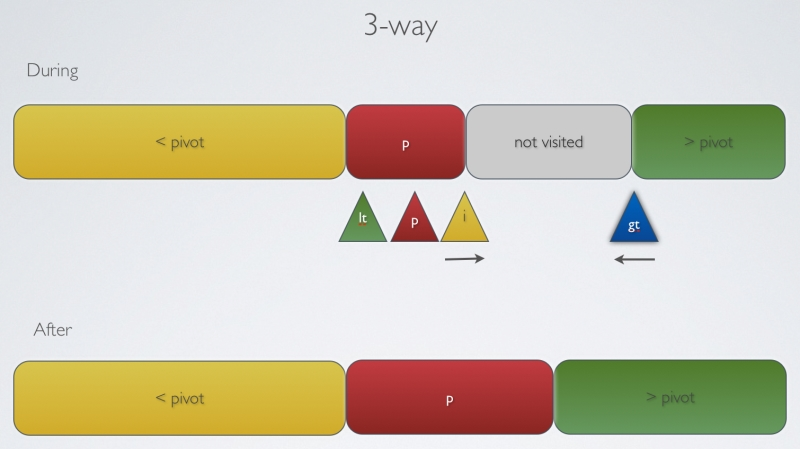双基准快速排序 转

• 基本排序：1222ms。

• 三路（Three-way）快速排序：1295ms（我是认真的！）。

• 双基准快速排序：1066ms。

• 基本排序：378ms。

• 三路快速排序：15ms。

• 双基准快速排序：6ms。

else if (pivot1==pivot2){
while (pivot1==pivot2 && lowIndex<highIndex){
lowIndex++;
pivot1=input[lowIndex];
}
}package basics.sorting.quick;

import static basics.sorting.utils.SortUtils.exchange;
import static basics.sorting.utils.SortUtils.less;
import basics.shuffle.KnuthShuffle;

public class QuickSortBasic {

public void sort (int[] input){

//KnuthShuffle.shuffle(input);
sort (input, 0, input.length-1);
}

private void sort(int[] input, int lowIndex, int highIndex) {

if (highIndex<=lowIndex){
return;
}

int partIndex=partition (input, lowIndex, highIndex);

sort (input, lowIndex, partIndex-1);
sort (input, partIndex+1, highIndex);
}

private int partition(int[] input, int lowIndex, int highIndex) {

int i=lowIndex;
int pivotIndex=lowIndex;
int j=highIndex+1;

while (true){

while (less(input[++i], input[pivotIndex])){
if (i==highIndex) break;
}

while (less (input[pivotIndex], input[--j])){
if (j==lowIndex) break;
}

if (i>=j) break;

exchange(input, i, j);

}

exchange(input, pivotIndex, j);

return j;
}

}package basics.sorting.quick;

import static basics.shuffle.KnuthShuffle.shuffle;
import static basics.sorting.utils.SortUtils.exchange;
import static basics.sorting.utils.SortUtils.less;

public class QuickSortDualPivot {

public void sort (int[] input){
//input=shuffle(input);
sort (input, 0, input.length-1);
}

private void sort(int[] input, int lowIndex, int highIndex) {

if (highIndex<=lowIndex) return;

int pivot1=input[lowIndex];
int pivot2=input[highIndex];

if (pivot1>pivot2){
exchange(input, lowIndex, highIndex);
pivot1=input[lowIndex];
pivot2=input[highIndex];
//sort(input, lowIndex, highIndex);
}
else if (pivot1==pivot2){
while (pivot1==pivot2 && lowIndex<highIndex){
lowIndex++;
pivot1=input[lowIndex];
}
}

int i=lowIndex+1;
int lt=lowIndex+1;
int gt=highIndex-1;

while (i<=gt){

if (less(input[i], pivot1)){
exchange(input, i++, lt++);
}
else if (less(pivot2, input[i])){
exchange(input, i, gt--);
}
else{
i++;
}

}

exchange(input, lowIndex, --lt);
exchange(input, highIndex, ++gt);

sort(input, lowIndex, lt-1);
sort (input, lt+1, gt-1);
sort(input, gt+1, highIndex);

}

}

2018/11/27
0
0

2016/06/06
1K
0

2018/07/20
0
0
iOS 算法~快速排序实现

//联系人:石虎QQ:1224614774 昵称:嗡嘛呢叭咪哄 一、概念: 快速排序: 是高快省的排序算法,在快速排序算法中，使用了分治策略。首先把序列分成两个子序列，递归地对子序列进行排序，直到整个序...

2017/12/23
0
0

2015/10/03
0
0

cesium调用天地图服务

25分钟前
4
0
Docker常用命令

_Change_
25分钟前
5
0
Spark ML使用DataFrame进行K-Means

1.前言 前一篇文章使用了RDD的方式,进行了K-Means聚类. 从Spark 2.0开始，程序包中基于RDD的API spark.mllib已进入维护模式.现在，用于Spark的主要机器学习API是软件包中基于DataFrame的API...

28分钟前
4
0

在面试中遇到美女面试官时，我们以为面试会比较容易过，也能好好表现自己技术的时候了。然而却出现以下这一幕，当美女面试官听说你使用过Redis时，那么问题来了。 👩面试官：Q1，你知道...

ccww_
32分钟前
5
0

36分钟前
3
0